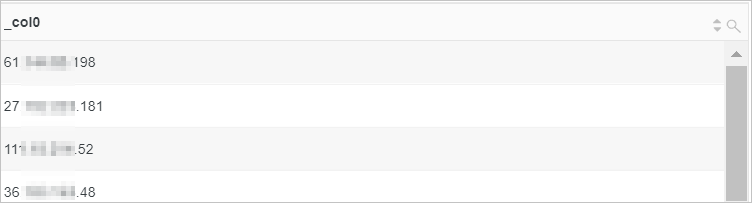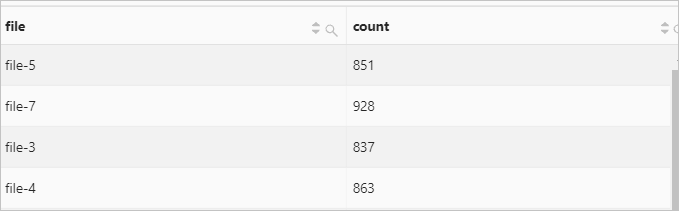This topic describes the syntax of conditional expressions and provides examples on how to use conditional expressions.

Expression Syntax Description
CASE WHEN statement CASE WHEN condition1 THEN result1

[WHEN condition2 THEN result2]

[ELSE result3]

END

Classifies data based on specified conditions.
IF function IF(condition, result1) If condition is evaluated to true, result1 is returned. Otherwise, null is returned.
IF(condition, result1, result2) If condition is evaluated to true, result1 is returned. Otherwise, result2 is returned.
COALESCE function COALESCE(expression1, expression2, expression3...) Returns the first non-null value in multiple expressions.
NULLIF function NULLIF(expression1, expression2) Evaluates whether the values of two expressions are the same. If the values are the same, null is returned. Otherwise, the value of the first expression is returned.
TRY function TRY(expression) Captures errors to ensure that Log Service can continue to query and analyze data.

## CASE WHEN statement

CASE WHEN statements are used to classify data.

### Syntax

``````CASE WHEN condition1 THEN result1
[WHEN condition2 THEN result2]
[ELSE result3]
END``````

### Parameters

Parameter Description
condition The conditional expression.
result1 The result that you want to return.

### Examples

• Example 1: Extract browser information from the value of the http_user_agent field. Then, classify the information into Chrome, Safari, and unknown types and calculate the number of page views (PVs) for the three types.
• Query statement
``````* |
SELECT
CASE
WHEN http_user_agent like '%Chrome%' then 'Chrome'
WHEN http_user_agent like '%Safari%' then 'Safari'
ELSE 'unknown'
END AS http_user_agent,
count(*) AS pv
GROUP BY
http_user_agent``````
• Query and analysis result• Example 2: Query the distribution of requests that are sent at different points in time.
• Query statement
``````* |
SELECT
CASE
WHEN request_time < 10 then 't10'
WHEN request_time < 100 then 't100'
WHEN request_time < 1000 then 't1000'
WHEN request_time < 10000 then 't10000'
ELSE 'large'
END AS request_time,
count(*) AS pv
GROUP BY
request_time``````
• Query and analysis result## IF function

The IF function is used to classify data. This function works in a similar manner to CASE WHEN statements.

### Syntax

• If condition is evaluated to true, result1 is returned. Otherwise, null is returned.
``IF(condition, result1)``
• If condition is evaluated to true, result1 is returned. Otherwise, result2 is returned.
``IF(condition, result1, result2)``

### Parameters

Parameter Description
condition The conditional expression.
result The result that you want to return.

### Examples

Calculate the ratio of requests whose status code is 200 to all requests.

• Query statement
``````* |
SELECT
sum(IF(status = 200, 1, 0)) * 1.0 / count(*) AS status_200_percentag``````
• Query and analysis result## COALESCE function

The COALESCE function is used to return the first non-null value in multiple expressions.

### Syntax

``COALESCE(expression1, expression2, expression3...)``

### Parameters

Parameter Description
expression The value of this parameter can be an expression of an arbitrary data type.

### Examples

Calculate the ratio of the expenses of the previous day to the expenses of the same day in the previous month.

• Query statement
``````* |
SELECT
compare("expenses of the previous day", 604800) AS diff
FROM  (
SELECT
COALESCE(sum(PretaxAmount), 0) AS "expenses of the previous day"
FROM log
)``````
• Query and analysis result• The value 6514393413.0 indicates the expenses of the previous day.
• The value 19578267596.0 indicates the expenses of the same day in the previous month.
• The value 0.33273594719539659 indicates the ratio of the expenses of the previous day to the expenses of the same day in the previous month.

## NULLIF function

The NULLIF function is used to check whether the values of two columns are the same. If the values are the same, null is returned. Otherwise, the value of the first expression is returned.

### Syntax

``NULLIF(expression1, expression2)``

### Parameters

Parameter Description
expression The valid scalar expression.

### Examples

Check whether the values of the client_ip and host fields are the same. If the values are not the same, the value of the client_ip field is returned.

• Query statement
``* | SELECT NULLIF(client_ip,host)``
• Query and analysis result## TRY function

The TRY function is used to capture errors to ensure that Log Service can continue to query and analyze data.

### Syntax

``TRY(expression)``

### Parameters

Parameter Description
expression The value of this parameter can be an expression of an arbitrary data type.

### Examples

If an error occurs when the regexp_extract function is invoked, the TRY function captures the error. This way, Log Service can continue to query and analyze data. The query and analysis result is returned.
• Query statement
``````* |
SELECT
TRY(regexp_extract(request_uri, '.*\/(file.*)', 1)) AS file,
count(*) AS count
GROUP BY
file``````
• Query and analysis result